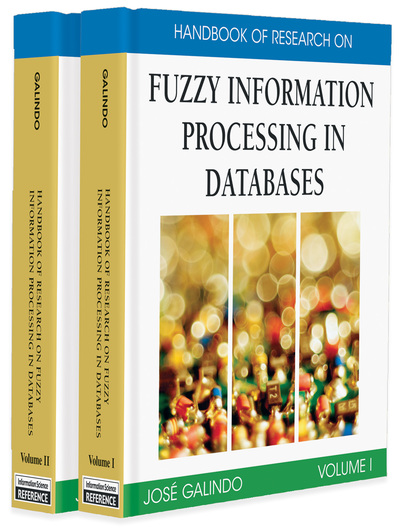# Incremental Discovery of Fuzzy Functional Dependencies

Shyue-Liang Wang (New York Institute of Technology, USA), Ju-Wen Shen (Chunghwa Telecom Lab, Taiwan) and Tuzng-Pei Hong (National University of Kaohsiung, Taiwan)
DOI: 10.4018/978-1-59904-853-6.ch024
Available
\$37.50
No Current Special Offers

## Abstract

Mining functional dependencies (FDs) from databases has been identified as an important database analysis technique. It has received considerable research interest in recent years. However, most current data mining techniques for determining functional dependencies deal only with crisp databases. Although various forms of fuzzy functional dependencies (FFDs) have been proposed for fuzzy databases, they emphasized conceptual viewpoints and only a few mining algorithms are given. In this research, we propose methods to validate and incrementally search for FFDs from similarity-based fuzzy relational databases. For a given pair of attributes, the validation of FFDs is based on fuzzy projection and fuzzy selection operations. In addition, the property that FFDs are monotonic in the sense that r1 ? r2 implies FDa(r1) ? FDa(r2) is shown. An incremental search algorithm for FFDs based on this property is then presented. Experimental results showing the behavior of the search algorithm are discussed.

## Key Terms in this Chapter

Incremental Search: Given a fuzzy relation r, a nonredundant COVER of FDa(r), and a new tuple t, it is the search for a COVER of new functional dependencies of FDa (r ? {t}) without processing r ? {t} directly.

Functional dependency: A functional dependency states that the value of an attribute is uniquely determined by the values of some other attributes.

Fuzzy Functional Dependency on Similarity-Based Fuzzy Relational Database: If t1.X and t2.X fall in the same equivalence class in X for a level value aX, then t1.A and t2.A also fall in the same equivalence class in A for another level value aA.

Similarity Relation: In general, a similarity relation is an interpropositional relation indicating that the events or states expressed in the propositions have a likeness of function or import. In this chapter, a similarity relation is a generalization of an equivalence relation that describes how similar two elements from the same domain are.

Equivalence Relation: It is a binary relation on a set X that is reflexive, symmetric, and transitive.

Equivalence Class: Given a set X and an equivalence relation ~ on X, the equivalence class of an element a in X is the subset of all elements in X that are equivalent to a.

Data Mining: Also called knowledge discovery in databases (KDD), it is the nontrivial extraction of implicit, previously unknown, and potentially useful information from data.

Similarity-Based Fuzzy Relational Data Model: It is a relation data model with two extensions. The first extension is that the tuple component tij selected from Dj is not constrained to be a singleton; instead, tij ? Dj and tij ? Ø. The second extension is that, for each domain Dj of a relation, a similarity relation sj is defined over the set elements.

## Complete Chapter List

Search this Book:
Reset